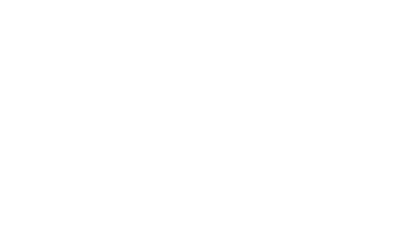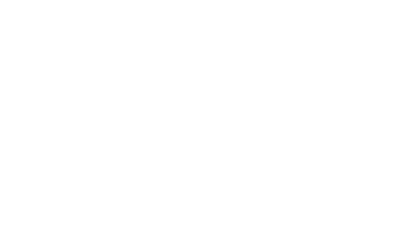## TURN

 Simultaneously rotates and crops an image to eliminate any background.

 USAGE: turn [-a angle] [-x xshift] [-y yshift] [-f format] infile outfilee USAGE: turn [-h or -help] -a .... angle ..... clockwise angle of rotation; 0<=float<=360; default=0 -x .... xshift .... xshift of image; integer; default=0 -y .... yshift .... yshift of image; integer; default=0 -f .... format .... format for the output; choices are aspect (a), ................... square (s) or maxarea (m); default=aspect PURPOSE: To simultaneously rotate and crop an image to eliminate any background. DESCRIPTION: TURN simultaneously rotate and crop an image to eliminate any background. Two methods are available: preserve the input image's w:h aspect ratio in the output or make the output square. ARGUMENTS: -a angle ... ANGLE of rotation. Values are floats in the range of 0 to 360 degrees. -x xshift ... XSHIFT of image. Values are integers (positive or negative). The default=0. -y xshift ... YSHIFT of image. Values are integers (positive or negative). The default=0. -f format ... FORMAT for the output image. The choices are: aspect (a), square (s) or maxarea (m). Format=aspect preserves the input image's w:h aspect ratio. Format=square makes the output square. Format=maxarea uses largest subsection possible for the output. The default=aspect. Requirement: IM 6.3.6-1 or higher due to the use of -set distort:viewport WxH+X+Y CAVEAT: No guarantee that this script will work on all platforms, nor that trapping of inconsistent parameters is complete and foolproof. Use At Your Own Risk.

### EXAMPLESAnimation: -a using 15 degree increments -f aspectAnimation: -a using 15 degree increments -f squareAnimation: -a using 15 degree increments -f maxareaWhat the script does is as follows: Computes the width and height and offsets of the rotated and cropped image to achieve either preservation of aspect ratio or a square result Uses viewport cropping with +distort SRT to rotate and crop the image This is equivalent to the following IM commands. w=`convert \$infile -ping -format "%w" info:` h=`convert \$infile -ping -format "%h" info:` [ \$w -ge \$h ] && mode="landscape" || mode="portrait" asang=`convert xc: -format "%[fx:abs(sin(\$angle*pi/180))]" info:` acang=`convert xc: -format "%[fx:abs(cos(\$angle*pi/180))]" info:` if [ "\$mode" = "landscape" ]; then a=\$w b=\$h wnum=`convert xc: -format "%[fx:\$a*\$b]" info:` hnum=`convert xc: -format "%[fx:\$b*\$b]" info:` else a=\$h b=\$w wnum=`convert xc: -format "%[fx:\$b*\$b]" info:` hnum=`convert xc: -format "%[fx:\$a*\$b]" info:` fi if [ "\$format" = "aspect" ]; then ww=`convert xc: -format "%[fx:\$wnum/(\$a*\$asang+\$b*\$acang)]" info:` hh=`convert \$infile -format "%[fx:\$hnum/(\$a*\$asang+\$b*\$acang)]" info:` elif [ "\$format" = "square" ]; then ww=`convert xc: -format "%[fx:\$b/(\$asang+\$acang)]" info:` hh=\$ww fi ww=`convert xc: -format "%[fx:floor(\$ww)]" info:` hh=`convert xc: -format "%[fx:floor(\$hh)]" info:` xoff=`convert xc: -format "%[fx:ceil((\$w-\$ww)/2)]" info:` yoff=`convert xc: -format "%[fx:ceil((\$h-\$hh)/2)]" info:` geometry=`printf "%dx%d%+d%+d" \$ww \$hh \$xoff \$yoff` convert \$infile -set option:distort:viewport \$geometry -virtual-pixel \$vp \ +distort SRT \$angle +repage \$outfile